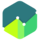COURSE

## Probability Concepts

In this course, you will learn about probability theory and its applications in measuring risk in investments.

• Common terminology of probability concepts
• Calculating probability of an event
• Unconditional and conditional probabilities
• Multiplication, addition and total probability rules
• Joint probabilities
• Conditional expectations in investment applications
• Covariance and Correlation
• Expected value, variance and standard deviation of an asset and a portfolio
• Bayes’ formula
• Solving counting problems using factorials, combinations and permutations

#### Course Resources

Study Notes for Probability Concepts
Member-only

#### Course Quizzes